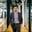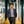Trusted answers to developer questions
Trusted Answers to Developer Questions

Related Tags

# What is the Recaman's sequence?Muhammad Ahmad

Grokking Modern System Design Interview for Engineers & Managers

Ace your System Design Interview and take your career to the next level. Learn to handle the design of applications like Netflix, Quora, Facebook, Uber, and many more in a 45-min interview. Learn the RESHADED framework for architecting web-scale applications by determining requirements, constraints, and assumptions before diving into a step-by-step design process.

### Overview

The Recaman's sequence is named after its inventor, Bernardo Recaman. It's a well-known sequence that is defined by the recurrence relation. We can define it by recursion.

### The recurrence relation

In the recurrence relation of the Recaman's sequence, at least two sequences are attributed. We form the sequence $a$n by taking $a$0 $= 0$ and letting the following:

### Conditions

Recurrence relations must fulfill the following conditions:

• Condition 1: Every term of the sequence must be positive.
• Condition 2: The absolute difference between the term and its preceding term equal the term number $n$.

In the recurrence relation, we subtract, or visit the previous term, if we can. Otherwise, we add, or visit next term. We'll understand this by seeing some initial terms.

Let's generate this sequence through C/C++ program.

• Program: Program to the $n$th number in Recaman's sequence
• Input: n = 10
• Output: The initial terms are 0, 1, 3, 6, 2, 7, 13, 20, 12, 21, 11, ...
arr(0) = 0if n > 0 and the number is not    already included in the sequence,        arr(n) = arr(n - 1) - n  //Visit the previous number in seqelse      arr(n) = arr(n - 1) + n //Visit the next number in seq
The pseudo-code for the recursive function

This pseudo-code is a function whose range is natural numbers, with $0$ as the first term. Specifically, $a(n)$ denotes the $(n+1)$th term. $0$ is already there.

// This program prints n-th number of the Recaman's sequence#include <iostream>using namespace std;// The function prints the first n terms of the Recaman's sequenceint rec_seq(int size){	// Create an array to store terms	int arr[size];	// The very first term of the sequence is always 0	arr = 0;	cout << arr<<" ,";    int i = 1;	int j = 0;	// Generate the rest of the terms using the recursive	// formula	while(i< size)	{		int curr = arr[i-1] - i;  //Visit the previous term on line 		int j;		while(j < i)		{			// If arr[i-1] - i is negative or the term already exists						if ((arr[j] == curr) || curr < 0)			{				curr = arr[i-1] + i; // Visit the next term				break;			}			j++;		}		arr[i] = curr;		cout << arr[i] << " ,";		i++;	}}// Driver codeint main(){	int size = 10;	cout << "First "<< size << " terms are: " ;	rec_seq(size);	cout << "......."<<endl;	return 0;}
A Raceman's sequence generator

### Explanation

The code chunk above generates the Recaman's sequence and saves it into an array of input size $n$. We only need to understand the following lines from the code.

• Line 13: The first term of the sequence is always $0$, that is, $a$0 $=$ $0$
• Line 20: This generates the previous number by arr[i - 1] - i.
• Line 27: This checks if the number generated by line 20 is already present in the array, or if it's a negative number.
• Line 29: If the number is negative or already present in the sequence, it generates the next number by arr[i - 1] + 1.

### Use case

We can use Recamán's sequence to secure 2D images by steganography.Steganography is the practice of concealing a message within another message or a physical object.

### Illustration

Neil Sloane has conjectured that every number eventually appears, but this has not been proved. The illustration below will give us an idea of how the algorithm produces a sequence by moving backward and forward on a number line.

Simulation of a Recaman's sequence

RELATED TAGS

CONTRIBUTORMuhammad Ahmad